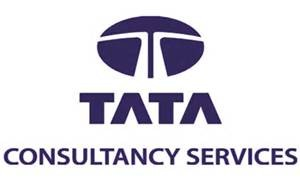11) Siva, Sathish, Amar and Praveen are playing cards. Amar is to the right of Sathish, who is to the right of Siva. Who is to the right of Amar?

12) Ferrari is leading car manufacturer. Ferrari S.P.A. is an Italian sports car. It has enjoyed great success. If Mohan’s Ferrari is 3 times faster than his old MERCEDES which gave him 35 km/ph if Mohan travelled 490 km in his Ferrari the how much time in hours he took?
Explanation:
Time = (Distance/Speed) = (490/35) Therefore the total time = time/3 = (490/35)/3 = 4.6666 Approximately 4.7

13) My flight takes off at 2 AM from a place at 18N 10E and landed 10 Hrs later at a place with coordinates 36N 70W. What is the local time when my plane landed?
Explanation:
Total change in degrees in east-west direction = 70 + 10 degrees =80 degrees, Time change due to the change in Longitude = 80*4 = 320 minutes = 5 hrs 20 min. According to the person the time would have been = 2 am + 10 hrs = 12 am. But according to the local time the time would be = 12 – 5.20 = 6 .40am

14) A class of 25 students took a science test. 10 students had an average (arithmetic mean) score of 80. The other students had an average score of 60. What is the average score of the whole class?
Explanation:
80 × 10 + 60 × 15 = 800 + 900 = 1700 Total number of students = 25 Average weight = 1700/25 The average score of the whole class is 68.

15) The cost of one pencil, two pens and four erasers is Rs.22 while the cost of five pencils, four pens and two erasers is Rs.32. How much will three pencils, three pens and three erasers cost?
Explanation:
x + 2y + 4z = 22 ——–>1 5x + 4y + 2z = 32 ——->2 multiply eqn (2) by 2 and substract from eqn(1) dividing by 3 we get 3x + 2y = 14———>3 multiplying eqn (1) by 5 and subtracting eqn (2) then divide by 6 we get y + 3z = 13———->4 Adding eqn (3) and (4) we get 3x + 3y +3z = 27

16) A is 20 percent more efficient than B. If the two persons can complete a piece of work in 60 days. In how many days A working alone can complete the work?
Explanation:
Ratio of time taken by A and B is 1: 1.2 i.e. 5:6 Let the time taken by A be 5x and by B be 6x , 1/5x + 1/ 6x = 1/60 => x =22. Time taken by A = 5 * 22 = 110

17) On a certain island, 5% of the 10000 inhabitants are one-legged and half of the others go barefooted. What is the least number of Shoes needed in the island?
Explanation:
In any case the one legged people will all require one shoe per head. From the remaining, half will go barefooted and therefore they need no shoes. And the rest will need two shoes per head. And this works out at one shoe per person for the others. Therefore for the whole population on the average one shoe per head

18) Look at this series: 14, 28, 20, 40, 32, 64, what number should come next?
Explanation:
This is an alternating multiplication and subtracting series: First, multiply by 2 and then subtract 8.

19) Two pipes A and B can fill a tank in 24 min. and 32 min. respectively. If both the pipes are opened simultaneously, after how much time B should be closed so that the tank is full in 18 minutes?
Explanation:
Let B be closed after x minutes. Then, part filled by (A + B) in x min. + part filled by an in (18 – x) min. = 1. X {1/24 + 1/32] + (18 – x) X 1/24 = 1 or 7x/96 + (18 – x)/24 = 1 7x + 4(18 -x) = 96 or x=8 Hence, B must be closed after 8 minutes

20) At a car park there are 100 vehicles, 60 of which are cars, 30 are vans and the remaining are Lorries. If every vehicle is equally likely to leave, find the probability of car leaving second if either a lorry or van had left first :
Explanation:
Let S be the sample space and A be the event of a van leaving first. n(S) = 100 n (A) = 30 Probability of a van leaving first: P (A) = 30/100 = 3/10 Let B be the event of a lorry leaving first. n (B) = 100 – 60 – 30 = 10 Probability of a lorry leaving first: P (B) = 10/100 = 1/10 If either a lorry or van had left first, then there would be 99 vehicles remaining, 60 of which are cars. Let T be the sample space and C be the event of a car leaving. n (T) = 99 n(C) = 60 Probability of a car leaving after a lorry or van has left: P(C) = 60/99 = 20/33